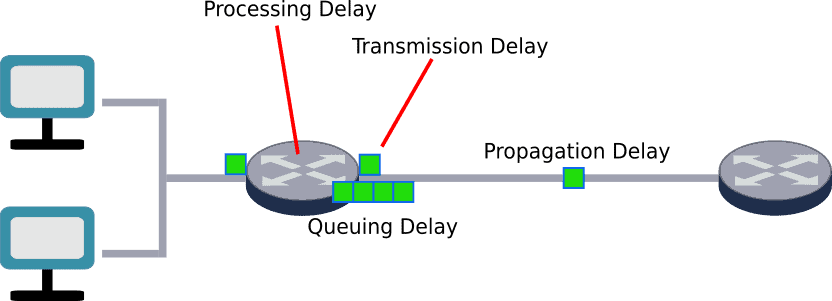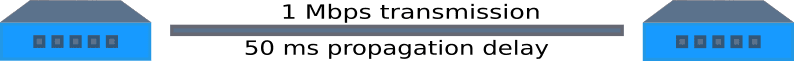## 1. Overview

In this tutorial, we’ll discuss network bandwidth, latency, packet time in detail. We’ll present numerical examples to calculate packet time using network latency and bandwidth.

## 2. Network Bandwidth

Network bandwidth represents the maximum amount of data we can transmit over a wireless or wired communication channel for a specified amount of time. Similarly, network throughput is the actual amount of data transmitted per unit of time in a network. Hence, the actual network throughput is always less than the network bandwidth.

In general, if a connection has significant bandwidth, the transmission of the data per unit would be high. Additionally, bandwidth is not the same as network speed. Hence, we can define network speed as the rate at which we process the data transmission. On the other hand, network bandwidth deals with the capacity of the communication channel.

When a communication link is full of traffic, the easiest option to fix is to increase the bandwidth of the network. However, network administrators and engineers are responsible for optimizing and monitoring the use of bandwidth in a network.

Network bandwidth is a limited resource. Therefore, it depends on the locations and network devices used for communication. Additionally, we measure network bandwidth in bits, kilobits, megabits. Modern devices such as 4K video-enabled TVs and video conference applications use high network bandwidth.

## 3. Delays in Network

There’re various factors that affect the time taken by a packet to reach the destination from a source in computer networks. Additionally, delays during the packet happen due to various factors, including packet loss, encryption, distance. There’re different delays in networking, including processing delay, queuing delay, transmission delay, and propagation delay:Processing delay () is a delay due to the processing of packet headers by routers. Delay from the routers is due to checking bit errors, figuring out the next-hop to which packet needs to be sent, and encryption operations. The second type of delay a packet can face is queuing delay (). It’s the time taken in routing queues. Queuing delay is the time data waits in the buffer of a router. If there is congestion in the network, there might be a significant queuing delay.

Transmission delay () is the time to push all the available data in a transmission medium or wire. We can calculate transmission delay (in a second) by dividing the number of bits () by the transmission rate ():Another delay a packet might face during data transmission is propagation delay (). It’s the time taken for a packet to cross the transmission medium. It depends on the distance () and speed of the packet (). Hence, propagation delay:## 4. Network Latency

Network latency () is the sum of all possible delays a packet can face during data transmission. We generally express network latency as round trip time (RTT) and measure in milliseconds (ms). Network delay includes processing, queuing, transmission, and propagation delays. Let’s look at the formula to calculate network latency:Depending on the networks and applications, the acceptable network latency varies. For example, applications including video conferences, VoIP calls, video streaming should have a low network latency to work efficiently. High network latency can significantly affect the performance of these applications. On the other hand, there’re applications like email where we can allow a high latency without highly affecting the performance of the application.

Round trip time (RTT) is the time it takes for a message to reach its destination from a source and then reach back from destination to source. Network ping time is very similar to round trip time. Round trip time is related to network latency. It’s not precisely double the network latency as there could be asymmetric latencies in both directions. Additionally, extra processing time at the destination is also included in round trip time.

We can calculate network throughput () using TCP receive window (W) and network round trip time (RTT)  which is related to latency:## 5. Example Calculations

### 5.1. Packet Time Calculation Based on Latency

Let’s calculate packet time using latency and bandwidth information. Consider a host and a switch. Let’s assume the transmission rate is 1 Mbps and propagation latency is 50 ms. Therefore, the question is, how much time will it take to transfer 1 KB of data packets assuming zero queuing and processing delays? Let’s find out:Here, we can utilize the network latency () formula discussed before:Additionally, we already discussed transmission delay and propagation delay formula in previous section. Hence, let’s revise the network latency () formula:Let’s put the values:Therefore, the total packet transfer time () would be 58 ms to transfer 1 KB data packets between the host and switch.

### 5.2. Packet Time Calculation Based on Throughput

Let’s discuss a practical approach to calculate actual throughput in an IP connection. An IP connection adjusts the calculated values for various factors, which slow down the data transfer rate. Therefore, we’ll consider the different delays we discussed before during the data transfer. Let’s discuss the details to calculate the time to transfer a certain amount of data given the link speed.

Firstly, we need to divide the number of bits to be transferred by link speed to get minimum theoretical time. We’re adding roughly 40% for TCP encoding overheads. Additionally, we add 22.5% of network congestion in this example. We assume data transfer is encrypted. Hence, we add a 12.5% processing delay.

Now the question is how much time will it take the data to transfer between location A and location B using a 20 Mbps link, to transfer 70 GB of data? Let’s find out.

First lets calculate the total packet transfer time () without considering any overhead:. Next, we also added 22.5% of network congestion. Hence, the packet transfer time after adjusting 22.5% extra for traffic:The packet transfer time after adjusting 12.5% for encrypted data:Hence, total time:Therefore, it takes roughly 15 hours to transfer 70 GB of data between locations A and B using a 20 Mbps link.

## 6. Conclusion

In this tutorial, we discussed the basics of network bandwidth, latency, packet time in detail. We presented numerical examples to calculate packet time using network latency and bandwidth.